# Digital Electronics MSQ

## 10 Questions MCQ Test Topic wise Tests for IIT JAM Physics | Digital Electronics MSQ

Description
Attempt Digital Electronics MSQ | 10 questions in 45 minutes | Mock test for IIT JAM preparation | Free important questions MCQ to study Topic wise Tests for IIT JAM Physics for IIT JAM Exam | Download free PDF with solutions
*Multiple options can be correct
QUESTION: 1

### Most demultiplexers facilitate which type of conversion?

Solution:

A demultiplexer sends a single input to multiple outputs, depending on the select lines. Demultiplexer converts single input into multiple outputs.

*Multiple options can be correct
QUESTION: 2

### Which of the following sets of values given below satisfy the Boolean relation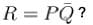Solution:

The realization of the algebraic expression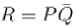in terms of logic gate is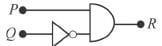Truth table.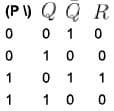Hence, (a), (c), (d)
The correct answers are: P = 0, Q = 0, R = 0, P = 1, Q = 0, R = 1, P = 1, Q = 1, R = 0

*Multiple options can be correct
QUESTION: 3

### The circuit shown in the figure can be used as :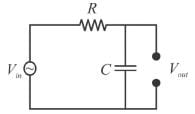Solution: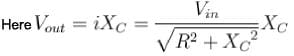Hence, it is an integrator and a low-pass filter.
The correct answers are: Integrator, Low-pass filter

*Multiple options can be correct
QUESTION: 4

A pulse is applied to each input of an exclusive OR gate. One pulse goes HIGH at t = 0 and goes back LOW at t = 1 ms. The other pulse goes HIGH at = 0.8 ms and goes back LOW at t = 3 ms. The output pulse can be described as follows :

Solution:

Output is HIGH when only one of the input is high.
The correct answers are: It goes HIGH at t = 0 and back LOW at t = 0.8 ms, It goes HIGH at t = 1 ms and back LOW at t = 3 ms

*Multiple options can be correct
QUESTION: 5

If the output of a logic gate is 0 only when all its inputs are at logic 1, then which of the following are/is not the corresponding gate?

Solution:

Truth table is,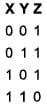This is truth table for NAND gate.

The gate whose output is low if and only if all inputs are high, is NAND.

The logic NAND gate is a combination of a digital logic AND gate and a NOT gate connected together in series.

A NAND gate (NOT-AND) is a logic gate which produces an output which is false only if all its inputs are true; thus its output is complement to that of an AND gate. A LOW (0) output results only if all the inputs to the gate are HIGH (1); if any input is LOW (0), a HIGH (1) output results.

*Multiple options can be correct
QUESTION: 6

The following circuit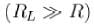does not performs the operation of.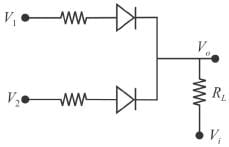Solution:

If either V1 or V2 is high, we get a high in output, hence it each like an OR gate.
The correct answers are: NAND gate for a negative logic system, AND gate for a positive logic system, AND gate for a negative logic system

*Multiple options can be correct
QUESTION: 7

Which of the following options is false for a two input XOR gate?

Solution: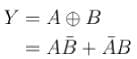The truth table for XOR gate is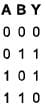(b), (c), (d).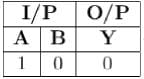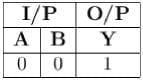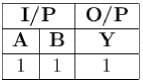*Multiple options can be correct
QUESTION: 8

Inputs given to a logic gate are A and B and its output is X. If A = 1, B = 0, then X = 1. What type of gate this could be?

Solution: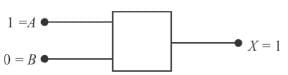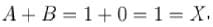clearly it follows OR gate.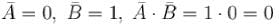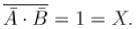Thus, it also follows NAND gate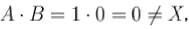it is an AND gate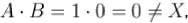it is not a NOR gate.
Therefore, the correct options are (b, c)
The correct answers are: NAND, OR

QUESTION: 9

The diagram of a logic circuit is given below. Which of the following represents the output F?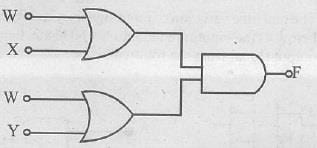Solution:

The gates (a) and (b) are  OR gates whereas c is an  AND gate. The boolean symbols for OR and AND gates are (+) and  (.) respectively.

Thus according to the logic circuit, the output of logic gates (a) and (b) will be  (W+X)  and  (W+Y)  respectively.

Now   F=(W+X). (W+Y)

⟹F=W+(X.Y)                  (using Boolean algebra)

*Multiple options can be correct
QUESTION: 10

A pulse is applied to each input of a 2 input NAND gate, one pulse goes high at t = 0 and goes back LOW at t = 1 ms. The other pulse goes high at f = 0.8 ms and goes Mark 0.00 out of 2.00 back LOW at t = 3 ms. The output pulse.

Solution:

A NAND gate is given by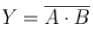Truth table is.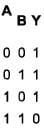It is low when both input signals are high otherwise it goes high.
The correct answers are: goes low at t = 0.8 ms, comes back high at t = 1 msUse Code STAYHOME200 and get INR 200 additional OFF Use Coupon Code